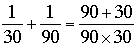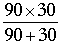SEARCH HOMEMath Central Quandaries & QueriesQuestion from bob, a student: T=(AB)/(A+B) using this formula t= time a,b representing each individual person i mow my lawn and it takes me 30 minutes my friend joe it takes 3 times as long,how long would it takes us together using this formulaHi Bob,

This is a question for which I would never use a formula. Formulas are much too easy to forget. For this and many similar problems I just remember to look at rates.

You mow the lawn in 30 minutes so your rate is 1 thirtieth of a lawn per minute (1/30 lawns/min). It takes Joe 3 times as long so it takes him 90 minutes and thus his rate is one ninetieth of a lawn per minute (1/90 lawns/min). Hence if you work together you can work at

1/30 + 1/90 lawns/min,

andlawns/minute.

But you want the number of minutes per lawn which is the reciprocal of lawns per minute so it will take youminutes/lawn.

Exactly what the formula says!

HarleyMath Central is supported by the University of Regina and The Pacific Institute for the Mathematical Sciences.